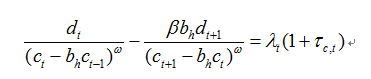# How to get the reasonable IRFs of fiscal volatility shocks

Hello everyone
I am trying to study the code file of 2015 AER paper “Fiscal Volatility Shocks and Economic Activity” by Fernández-Villaverde et al… When I run the code file “main_code_FGKR.mod” which provided by the author in AER web,I found that the model equations in the code file are not the same as those in the paper,for example, in the paper, the FOC of private consumption is, but in the code file, this equation is written as exp(dt)/(exp(ct)-habitexp(ct(-1))/exp(gzt))^ome - exp(dt(1))habitbet/(exp(ct(1))exp(gzt(1))-habitexp(ct))^ome= exp(lamt)(1+tauct) , according to this expression, the FOC of private should be, why does the author add the At in the code file? and how to introduce the “At” for other endogenous variables? Besides, there are two problems when I am trying to run the code file:
(1) When I run the “main_code_FGKR.mod”, the matlab could not get the results, and it shows as follows:
The function ‘RunDynarePruning’ corresponding to the input parameter of ‘struct’ is not defined.

Error author_maincode_practise (line 707)
outDynare = RunDynarePruning(optPruning,oo_,M_,f_11);

Error dynare (line 180)
Evalin(‘base’,fname) ;

How should I solve this problem?
(2) I read a lot of related topics in this forum, and I saw that the professor Jpfeifer recommended third-order algorithms in other posts, then I changed the code file by deleting section of " 5. Run Dynare with pruning" in the code file, and use stoch_simul(order = 3, pruning,k_order_solver,noprint,irf=40) directly to get the IRFs, but the IRFs are very weird, IRFs are very different from those in paper, why did I get different results? And how to get the same results as the author?

Can I get any help? thank a lot!
I have uploaded the author’s code and the weird IRFs .main_code_FGKR.mod (21.8 KB)
IRFs_capital tax volatility shock.pdf (97.3 KB)

It’s not my code, so I don’t have deeper insights. A couple of thoughts;

1. The A_t is related to a non-stationary technology process. To enter equations into Dynare, one needs to detrend the model variables. When variables with different timing appear, one gets the growth rates of technology as seems to be the case here.
2. Regarding the `RunDynarePruning` you need to check whether you get all the files or whether they require an external toolkit like Andreasen’s pruning toolkit.